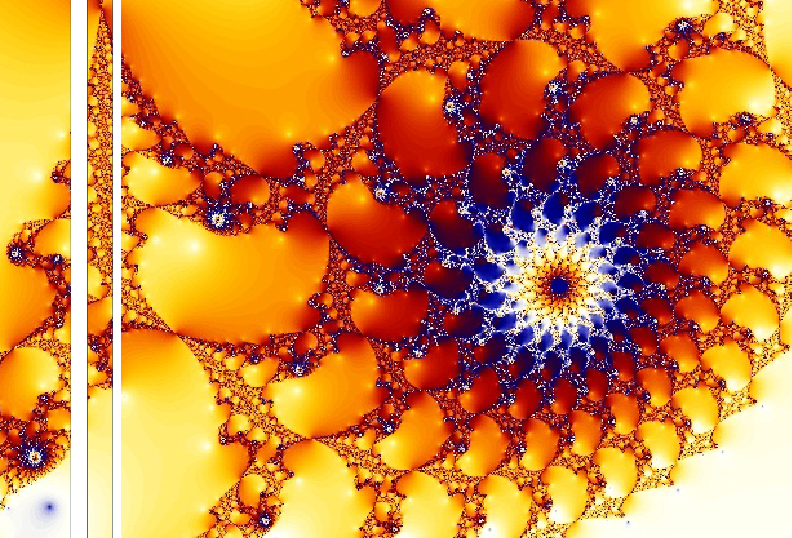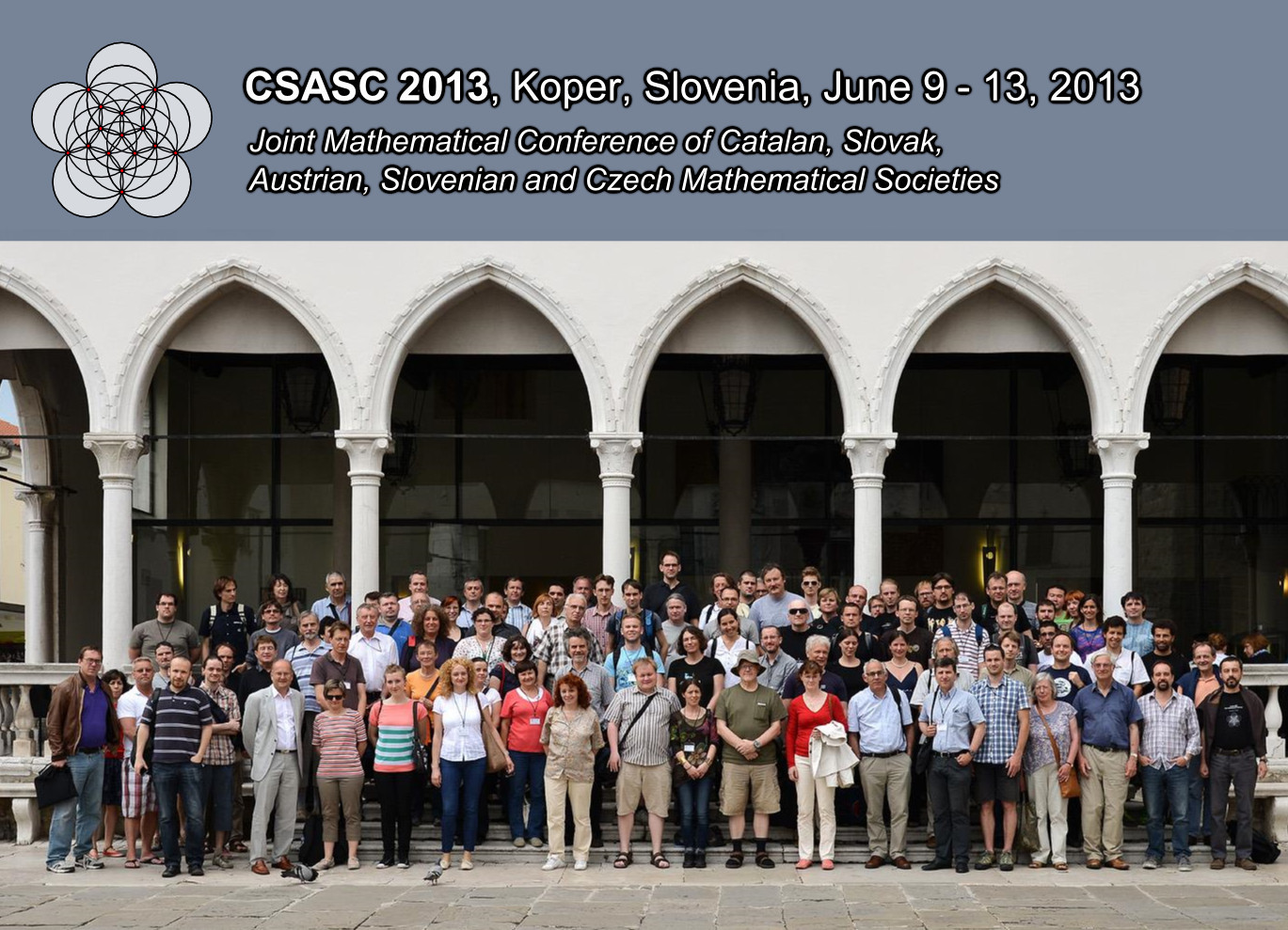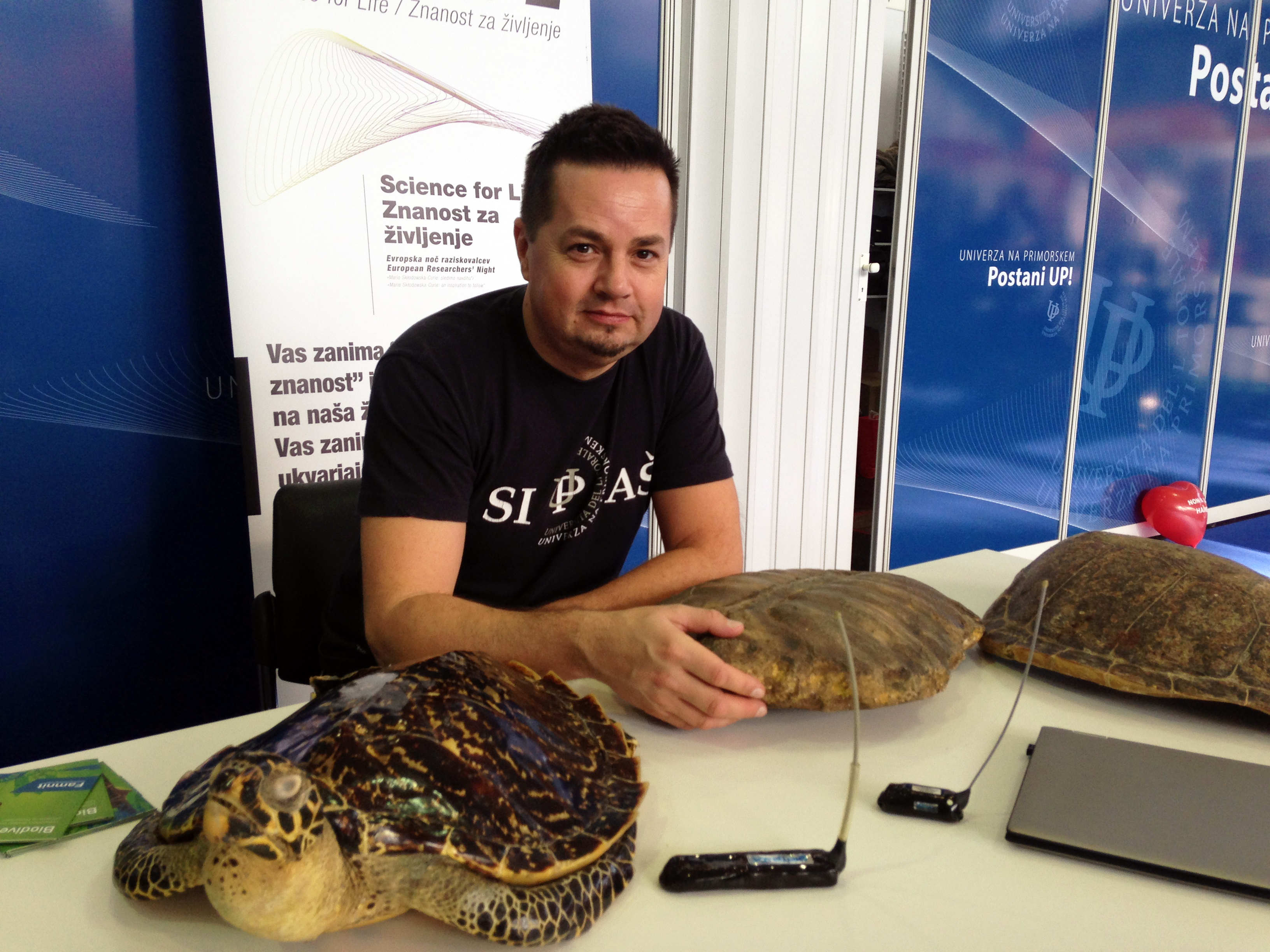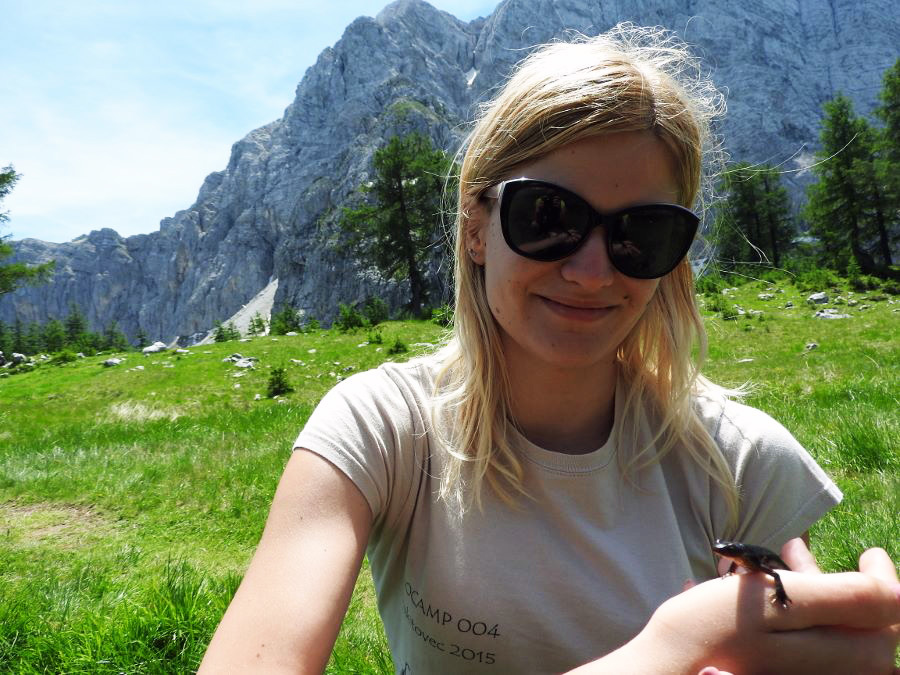Univerza na Primorskem Fakulteta za matematiko, naravoslovje in informacijske tehnologijeSI | EN# Raziskovalni matematični seminar - Arhiv

 2021 2020 2019 2018 2017 2016 2015 2014 2013 2012 2011 2010
 1 2 3 4 5 6 7 8 9 10 11 12
Datum in ura / Date and time: 31.5.21
(10:00 -- 11:00)
Predavalnica / Location: Zoom
Predavatelj / Lecturer: Michael Vyalyi (HSE University , Russia)
Naslov / Title: Re-pairing brackets
Vsebina / Abstract:

The re-pairing problem is a recently discovered combinatorial problem concerning Dyck words. We have first identified the re-pairing problem when studying an open question in automata theory, the complexity of translation of one-counter automata (OCA) on finite words into Parikh-equivalent nondeterministic finite automata (NFA). But it appears that the re-pairing problem has natural connections with other combinatorial problems arising in analysis of weak models of computation (e.g., black-and-white pebble games). We hope that more relations will be discovered in future.

In the talk I will define the problem and give a survey of known results about it.

This is joint work with D. Chistikov.

Join Zoom Meeting Here!

Datum in ura / Date and time: 24.5.21
(10:00 -- 11:00)
Predavalnica / Location: Zoom
Predavatelj / Lecturer: Matjaž Krnc (UP FAMNIT, Slovenia)
Naslov / Title: Shifting any path to an avoidable one
Vsebina / Abstract:

A vertex$v$ in a graph$G$ is avoidable if every induced path on three vertices with middle vertex$v$ is contained in an induced cycle. Dirac's classical result from 1961 on the existence of simplicial vertices in chordal graphs is equivalent to the statement that every chordal graph has an avoidable vertex. Beisegel, Chudovsky, Gurvich, Milani\v{c}, and Servatius (2019) generalized the notion of avoidable vertices to \emph{avoidable paths}, and conjectured that every graph that contains an induced$k$-vertex path also contains an avoidable induced$k$-vertex path; they proved the result for$k = 2$. The case$k = 1$ was known much earlier, due to a work of Ohtsuki, Cheung, and Fujisawa in 1976. The conjecture was proved for all$k$ in 2020 by Bonamy, Defrain, Hatzel, and Thiebaut. This result generalizes the mentioned results regarding avoidable vertices, as well as a result by Chv\'atal, Rusu, and Sritharan (2002) suggested by West on the existence of simplicial$k$-vertex paths in graphs excluding induced cycles of length at least$k+3$.

In this talk we discuss an adaptation of the approach by Bonamy et al.~from a reconfiguration point of view. We say that two induced$k$-vertex paths are \emph{shifts} of each other if their union is an induced path with$k+1$ vertices and show that in every graph, every induced path can be shifted to an avoidable one. We also present an analogous result about paths that are not necessarily induced, where an efficient reconfiguration sequence relies on the properties of depth-first search trees.

Join Zoom Meeting Here!

Datum in ura / Date and time: 17.5.21
(10:00 -- 11:00)
Predavalnica / Location: Zoom
Predavatelj / Lecturer: Blas Fernández (UP IAM, Slovenia)
Naslov / Title: On 2-Y-homogeneous and almost 2-Y-homogeneos distance-biregular graphs
Vsebina / Abstract:

Let G denote a distance-biregular graph with bipartite parts Y and Y’. Let D denote the eccentricity of vertices in Y. Given a vertex z, let \Gamma_i(z) denote the set of all vertices which are at distance i from z. For vertices x and y, let \Gamma_{i,j}(x,y) denote the collection of all vertices which are at distance i from x and at distance j from y.

In this talk, we will show necessary and sufficient conditions on the intersection array of G for which the given graph has one of the following two combinatorial structures:

1. for all i (1 \leq i \leq D-2) and for all x\in Y, y\in \G_2(x) and z \in \G_{i,i}(x,y) the number of vertices in \G_{1,1}(x,y) which are at distance i-1 from z is independent of the choice of x,y and z.

2. for all i (1 \leq i \leq D-1) and for all x\in Y, y\in \G_2(x) and z \in \G_{i,i}(x,y) the number of vertices in \G_{1,1}(x,y) which are at distance i-1 from z is independent of the choice of x,y and z.

Distance-biregular graphs with the previous combinatorial structures are called almost 2-Y-homogeneous and 2-Y-homogeneous, respectively. Several examples will also be presented. This is joint work with Safet Penjić.

Join Zoom Meeting HERE!

Everyone is welcome and encouraged to attend.

Datum in ura / Date and time: 10.5.21
(10:00 -- 11:00)
Predavalnica / Location: Zoom
Predavatelj / Lecturer: Slobodan Filipovski (UP FAMNIT)
Naslov / Title: On the clique number and Mantel/Turan theorems
Vsebina / Abstract:

Let G=(V,E) be a finite undirected graph with vertex set V(G) of order |V(G)|=n and edge set E(G) of size |E(G)|=m. Let d_{1}\geq d_{2}\geq...\geq d_{n} be the degree sequence of the graph G.

A clique in a graph G is a complete subgraph of G.  A clique is called maximum if it has maximum cardinality.
The clique number of a graph G, denoted \omega(G), is the order of a maximum clique of G.

In 1907 Mantel proved that a triangle-free graph with n vertices can contain at most  \$lfloor \frac{n^{2}}{4}\rfloor edges. In 1941 Tur\' an generalized Mantel's result to graphs not containing cliques of size r by proving that graphs of order n that contain no induced K_{r} have at most (1-\frac{1}{r-1}) \frac{n^{2}}{2} edges.

In this talk, we present new lower bounds for the clique number \omega(G). Moreover, we obtain a new bound for the maximum number of edges in a K_{r}-free graph G of order n, with minimum degree d_{n}, and
maximum degree d_{1}.

The results are based on elementary inequalities applied to the degree sequence d_{1}\geq d_{2}\geq\ldots \geq d_{n}.

We are looking forward to meeting at the video conference.

Join Zoom Meeting HERE!

Datum in ura / Date and time: 3.5.21
(10:00 -- 11:00)
Predavalnica / Location: Zoom
Predavatelj / Lecturer: Tomaž Pisanski (UP FAMNIT and UL FMF).
Naslov / Title: Embeddings of Action Graphs
Vsebina / Abstract:

In the literature, one can find at least three different genus parameters associated with a finite group: genus, symmetric genus, and strong symmetric genus. While the genus of a group is defined in terms of the (undirected, non-edge-colored) Cayley graphs, plain graphs are not adequate for modeling symmetric and strongly symmetric embeddings and thus cannot be used directly for determining the symmetric and strong symmetric genus of a group. We propose (generalized) action graphs to model such embeddings. Although action graphs are a wider class of edge-colored, partially directed graphs than Cayley color (di)graphs, the idea of symmetric and strongly symmetric embedding can be applied to them. In addition, we present some applications of action graphs.

This is joint work with Thomas W. Tucker.

We are looking forward to meeting at the video-conference.

Join Zoom Meeting HERE!